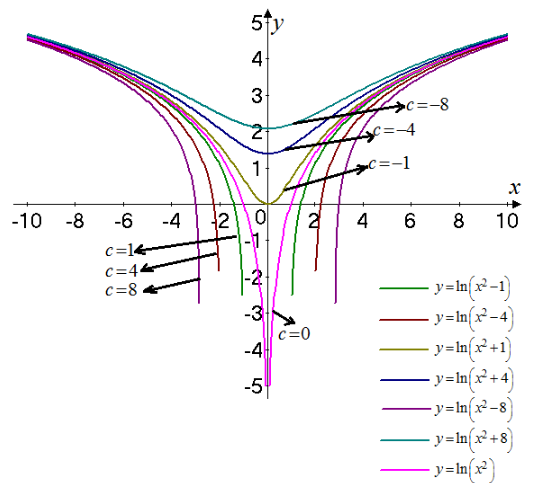# The graph for the member of the family of given functions for several values of c and then determine how the graph changes.### Single Variable Calculus: Concepts...

4th Edition
James Stewart
Publisher: Cengage Learning
ISBN: 9781337687805### Single Variable Calculus: Concepts...

4th Edition
James Stewart
Publisher: Cengage Learning
ISBN: 9781337687805

#### Solutions

Chapter 1, Problem 29RE
To determine

## To find: The graph for the member of the family of given functions for several values of c and then determine how the graph changes.

Expert Solution

The required graph is shown in Figure 1 and the graph is deeper at x=0 as the value of c increases.

### Explanation of Solution

Given:

The given function is f(x)=ln(x2c) .

Calculation:

Consider the given functions equations,

f(x)=ln(x2c)

The graph for the given function for the different values of c is shown in Figure 1Figure 1

The graph shows it is seen that for c<0 the function is defined at every point and as the value of c increases the dip of the graph at x=0 increases.

Consider the graph for c0 .

limx±cln(x2c)=

Thus, the graph asymptotes at x=c .

### Have a homework question?

Subscribe to bartleby learn! Ask subject matter experts 30 homework questions each month. Plus, you’ll have access to millions of step-by-step textbook answers!